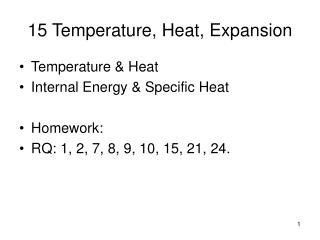DownloadDownload Presentation15 Temperature, Heat, Expansion

# 15 Temperature, Heat, Expansion

Télécharger la présentation## 15 Temperature, Heat, Expansion

- - - - - - - - - - - - - - - - - - - - - - - - - - - E N D - - - - - - - - - - - - - - - - - - - - - - - - - - -
##### Presentation Transcript

1. 15 Temperature, Heat, Expansion • Temperature & Heat • Internal Energy & Specific Heat • Homework: • RQ: 1, 2, 7, 8, 9, 10, 15, 21, 24.

2. Temperature • T ~ average kinetic energy • T increase = increase in speed of molecules, e.g. hammered metal increases in temperature. • liquids, gases, and solids usually expand when T increased

3. Water Expansion • Water expands from 4°Cto 100°C (as does most materials) • However, water contracts when warmed from 0°C to 4°C. (transient ice melting)

4. What happens to the hole size?

5. Heat • ‘heat’ is a transfer of thermal energy due to temperature difference • Examples: • ice in warm liquid: heat flows from liquid to the ice • warm liquid is put in a refrigerator: heat flows from drink to air and refrigerator • //

6. Internal Energy • the total of all molecular energies, kinetic plus potential, that are internal to a substance. It is ~ (mass) x (temperature). • Heat ≠ Internal Energy, • however, for a thermal-only process, • Heat = D(Internal Energy)

7. mixing: ‘hot’ and ‘cold’ = ‘warm’ • for liquids & solids: heat ~ (mass)x(temperature change) • when ‘hot’ and ‘cold’ are mixed, heat lost by ‘hot’ = heat gained by ‘cold’ • Ex. 1kg water at 0°C is mixed with 1kg water at 20°C: • (1kg)(T - 0) = (1kg)(20 – T)T = 20 – T 2T = 20T = 10°C

8. more mixing • Ex. 2kg water at 40°C is mixed with 1kg water at 20°C: • (1kg)(T - 20) = (2kg)(40 – T)T – 20 = 80 – 2T 3T = 100T = 100/3 = 33.3°C

9. Calories and Joules • 1 Calorie = 1000 calories • 1 calorie = 4.18 joule • Calorie is the common food unit. • Btu’s are also used, e.g. gas range

10. Specific Heat • the specific heat of a substance is the quantity of heat required to raise the temperature of 1kg of a substance 1°C. //

11. specific heat • c = Q/mDT [J/(kg·K)] • heat needed per kg to raise temperature by 1 degree C or K. • slope warming water = DT/Q = 1/(mc)

12. example c’s • in J/(kg-C): • aluminum 920 • copper 390 • ice 2100 • water 4186

13. Example: • A student wants to check “c” for an unknown substance. She adds 230J of heat to 0.50kg of the substance. The temperature rises 4.0K.

14. summary • T ~ avg. KE • most substances undergo thermal expansion (note the water exception) • heat is an exchange of thermal energy • specific heat = heat needed to raise temp. of 1kg by 1C (substance dependent)

15. Thermodynamics • flow and effect of thermal energy on matter (solids, liquids, gases) • temperature and internal energy • heat engines